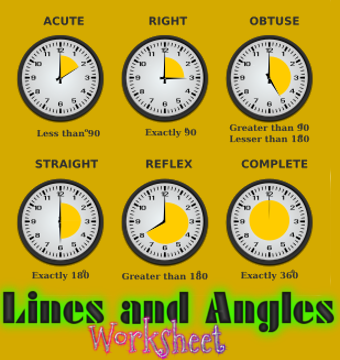## Lines and Angles (Worksheet)

Directions: Fill in the blanks to make the statements true.

1. If sum of measures of two angles is 90°, then the angles are _________.

3. If the sum of measures of two angles is 180°, then they are _________.

3. A transversal intersects two or more than two lines at _________ points.

If a transversal intersects two parallel lines, then (Q.4 to 7).

4. Sum of interior angles on the same side of a transversal is __________

5. Alternate interior angles have one common _______________

6. Corresponding angles are on the _______________ side of the transversal.

7. Alternate interior angles are on the ___________ side of the transversal.

8. Two lines in a plane which do not meet at a point anywhere are called ____________ lines.

9. Two angles forming a __________ pair are supplementary.

10. The supplement of an acute is always __________ angle.

11. The supplement of a right angle is always _________ angle.

12. The supplement of an obtuse angle is always _________ angle.

13. In a pair of complementary angles, each angle cannot be more than _________90°.

14. An angle is 45°. Its complementary angle will be __________ .

15. An angle which is half of its supplement is of __________.

Answers:
1. Complementary
2. Supplementary
3. Distinct
4. 180°
5. Arm
6. Same
7. Opposite
8. Parallel
9. Linear
10. Obtuse
11. right
12. acute
13. 90°
14. 45°
15. 60°

☛See also:

Ch 1 - Integers (NCERT Ex 1.2)

Ch 1 - Integers (NCERT Ex 1.3)

Ch 2 - Decimals - Multiplication and Division (Worksheet)
Ch 2 - Fractions and Decimals - NCERT Exercise Answers 2.6

Ch 3 - Data Handling (NCERT Ex 3.4)

CH 4 - Simple Equations (NCERT Ex 4.4)
CH 4 - Simple Equations (Worksheet)

CH6 - Lines and Angles (Worksheet)

CH8 - Comparing Quantities (Ratio and NCERT Ex 8.1)
CH8 - Comparing Quantities (Percentage and NCERT Ex 8.2)
CH8 - Comparing Quantities(NCERT Ex 8.3)

Chapter 12 - Algebraic Expressions (Revision Sheet)
Chapter 12 - Algebraic Expressions - NCERT Solution 12.1

Chapter 13: Exponents and Powers (MCQs)

Rules of Divisibility (Another post on Divisibility Rules)
Maths Challenges
Maths PSA Quiz (2016)
Maths SA2 Sample Question Paper (2016-17)
Maths SA2 Sample Question Paper (2016-17) - set 2
Maths SA2 Sample Question Paper (2016-17) - set 3#### Post a Comment

We love to hear your thoughts about this post!